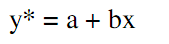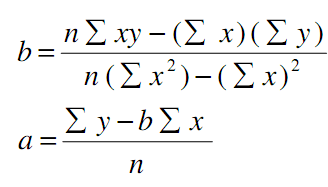# Regression Analysis

Regression analysis is a quantitative research method which is used when the study involves modelling and analysing several variables, where the relationship includes a dependent variable and one or more independent variables. In simple terms, regression analysis is a quantitative method used to test the nature of relationships between a dependent variable and one or more independent variables.

The basic form of regression models includes unknown parameters (β), independent variables (X), and the dependent variable (Y).

Regression model, basically, specifies the relation of dependent variable (Y) to a function combination of independent variables (X) and unknown parameters (β)

Y ≈ f (X, β)

Regression equation can be used to predict the values of ‘y’, if the value of ‘x’ is given, and both ‘y’ and ‘x’ are the two sets of measures of a sample size of ‘n’. The formulae for regression equation would beWhere,Do not be intimidated by visual complexity of correlation and regression formulae above. You don’t have to apply the formula manually, and correlation and regression analyses can be run with the application of popular analytical software such as Microsoft Excel, Microsoft Access, SPSS and others.

Linear regression analysis is based on the following set of assumptions:

1. Assumption of linearity. There is a linear relationship between dependent and independent variables.

2. Assumption of homoscedasticity. Data values for dependent and independent variables have equal variances.

3. Assumption of absence of collinearity or multicollinearity. There is no correlation between two or more independent variables.

4. Assumption of normal distribution. The data for the independent variables and dependent variable are normally distributed

My e-book, The Ultimate Guide to Writing a Dissertation in Business Studies: a step by step assistance offers practical assistance to complete a dissertation with minimum or no stress. The e-book covers all stages of writing a dissertation starting from the selection to the research area to submitting the completed version of the work within the deadline. John Dudovskiy[]